• 之前再看pr DQN的时候，还有pg的时候都会说到重要性采样，尽管目前我对其应用还有一些疑惑。 但是今天看了一篇博客，大概算是看懂了。转自https://blog.csdn.net/wangpeng138375/article/details/74645637 下面是...
之前再看pr DQN的时候，还有pg的时候都会说到重要性采样，尽管目前我对其应用还有一些疑惑。

但是今天看了一篇博客，大概算是看懂了。转自https://blog.csdn.net/wangpeng138375/article/details/74645637

下面是博客内容：简单直观

因为我是做强化学习的，还有一个强化学习IS的连接https://blog.csdn.net/philthinker/article/details/76038513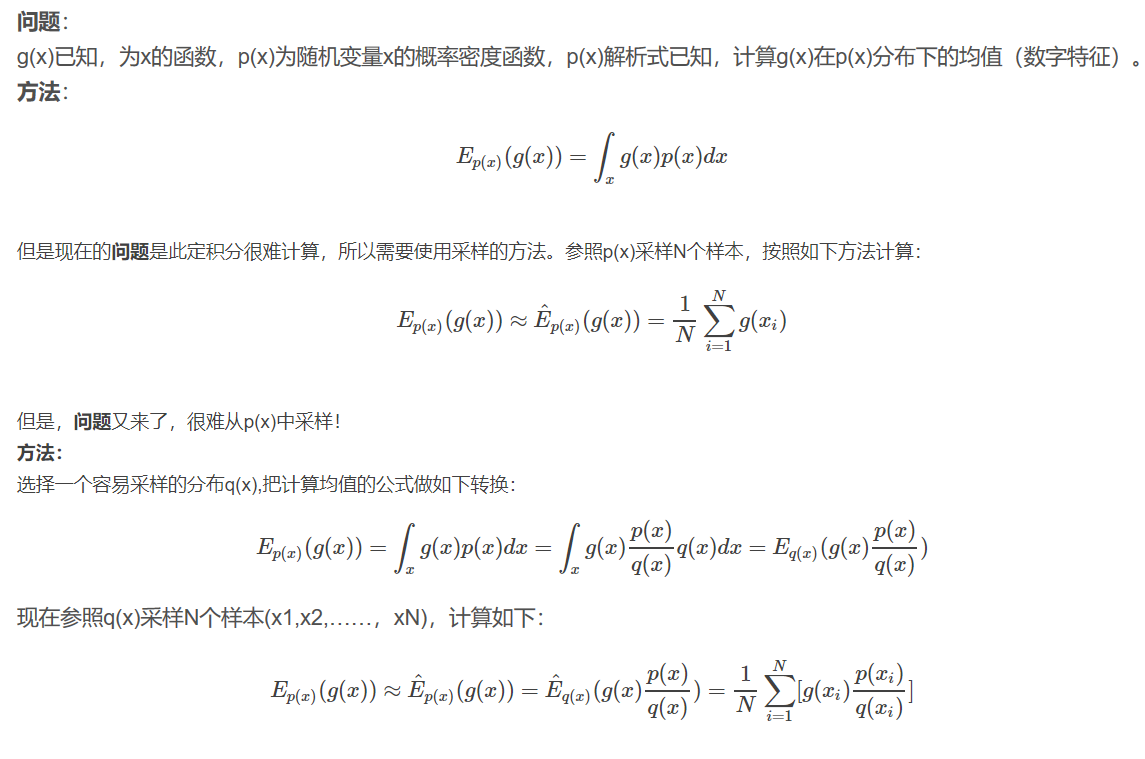展开全文• 重要性采样和多重重要性采样在路径追踪中的应用1 蒙特卡洛路径追踪简要回顾1.1 算法主要流程1.2 半球面均匀采样方法2 重要性采样的运用2.1 简单例子与基本概念2.2 路径追踪中的重要性采样2.2.1 Cosine-weighted 半球...
重要性采样和多重重要性采样在路径追踪中的应用1 蒙特卡洛路径追踪简要回顾1.1 算法主要流程1.2 半球面均匀采样方法2 重要性采样的运用2.1 简单例子与基本概念2.2 路径追踪中的重要性采样2.2.1 Cosine-weighted 半球采样2.2.2 BRDF采样3 多重重要性采样的运用总结：Refernce：
在之前的文章中，我们介绍了利用蒙特卡洛路径追踪来解渲染方程得到一个近似解的方法，但使用朴素的均匀采样来求解积分往往会导致比较大的方差和缓慢的收敛速度。因此将积分分为直接光照（对光源的直接采样）和间接光照的求解一定程度上缓解了这个问题，但依然有很大的提升空间。在本文中会继续深入该问题，讲解如何使用重要性采样以及多重重要性采样的方法提升收敛速度。
全文大致分为三章，第一章会简要回顾一下蒙特卡洛路径追踪的做法，并补充一些之前没有提到过的具体的均匀采样半球面的方法。第二章会介绍重要性采样的方法来加快收敛速度。第三章则会在第二章的基础之上，将多种重要性采样方法结合在一起，得到不同采样分布的优点使得路径追踪算法更加的robust。
1 蒙特卡洛路径追踪简要回顾
1.1 算法主要流程
所有的全局光照算法其实都是围绕着如何求解渲染方程：
$L_{o}\left(p, \omega_{o}\right)=L_{e}\left(p, \omega_{o}\right)+\int_{\Omega^{+}} L_{i}\left(p, \omega_{i}\right) f_{r}\left(p, \omega_{i}, \omega_{o}\right)\left(n \cdot \omega_{i}\right) \mathrm{d} \omega_{i}$
着色点$p$向$\omega_o$发出的光线由两部分组成，一部分是本身的自发光(一般只有光源才有，其他物体为0)，另一部分由法线所在半球的各个方向上的光线共同贡献得到。
路径追踪的方法也非常的直观，即每次碰撞到物体之后都去采样一条可能的scatter的方向，然后递归这个过程直到光线逃逸出场景，或达到终止条件，又或者击中光源形成一条真正可行的光路。简单图示如下：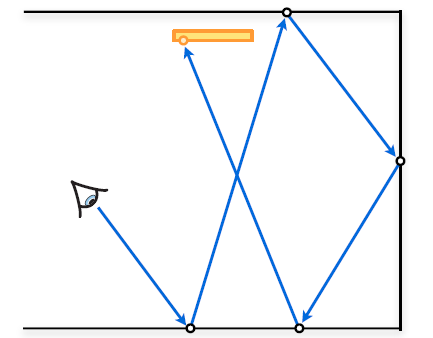了解了基本的路径追踪的过程，那么伪代码也就比较好写了：
Color color(Point p, Direction w, int depth)
{
if depth <= 0:
return Le(p, -w);

// 递归采样
w' = uniform sampling from hemisphere;
Point hit_point = trace(p, w');

// 逃逸出场景
if not hit something:
return 0;

return Le(p, -w) + BRDF * color(hit_point, w', depth-1) * cos / pdf;
}

当然以上的伪代码只是进行一次路径追踪的过程，蒙特卡洛路径追踪是利用多次路径追踪的结果求均值从而不断逼近真实解的一个过程，本质上就是蒙特卡洛积分：
$L_{o}\left(p, \omega_{o}\right) \approx \frac{1}{N} \sum_{i=1}^{N} \frac{L_{i}\left(p, \omega_{i}\right) f_{r}\left(p, \omega_{i}, \omega_{o}\right)\left(n \cdot \omega_{i}\right)}{p\left(\omega_{i}\right)}$
(具体的蒙特卡洛路径追踪的内容可以参考之前的文章，这里就简单的回顾一下)
1.2 半球面均匀采样方法
阅读上一小节的伪代码，可以看到在每次采样下一次光线的方向的时候，是对半球面进行均匀采样的，那么具体是如何做到的呢？为此，我们首先要介绍一下如何从一个给定的概率分布$pdf(x)$进行采样的方法。
问题描述: 设随机变量$X\sim pdf(x)$，记累积分布函数$F_X(x) = P(X \leq x)$，现希望从该概率分布中进行采样。
方法: 假设随机变量$Y\sim U（0,1）$，不难得出该满足均匀分布的随机变量的累积分布函数为$P(Y\leq y) = y$。现构造另一随机变量$Z=F^{-1}_X(Y)$，则
$P\left(F_{X}^{-1}(Y) \leq x\right)=P\left(Y \leq F_{X}(x)\right)=F_{X}(x)$
因此新构造出来的随机变量$Z$恰好符合随机变量$X$的分布，因此想要对随机变量$X$进行采样，可以转化为对随机变量$Z$进行采样，而对后者进行采样是颇为直接的，只用两步：
1.在(0,1)之内进行均匀采样得到$y_i$
2.再对其进行$F^{-1}_X(y_i)$的逆分布函数变换即可。
上述方法一般被称为逆分布函数方法，虽然只介绍了对一维随机变量的采样，但拓展到高维也是比较直接的，假设现在要生成二维随机变量$(\theta,\phi) \sim pdf(\theta,\phi)$，则可以先根据边缘概率密度$pdf(\theta)$生成$\theta_i$的采样，再根据条件概率密度$pdf(\phi|\theta)$生成$\phi_i$的采样即可，更高维也类似，不再详述。
好了，经过以上的介绍已经明白了如何从一个给定的分布中进行采样，那么接下来就应该回归到本节的标题，如何对半球进行均匀采样？
因为目标是对半球进行均匀采样，所以立体角满足的概率密度$pdf(\omega)$必然是一个常数，所以有：
\begin{aligned} & \int_{\Omega^{+}} pdf(\omega) \mathrm{d} \omega = 1\\ & \space\space \space\space\space pdf(\omega) = 1/2\pi \end{aligned}
现在的问题是虽然知道了立体角的概率密度，但没有办法从立体角转化到三维坐标，因此这里利用$d\omega = sin\theta d\theta d\phi$，将立体角转化为球面坐标，如果能对单位球面坐标$(\theta,\phi)$进行采样，自然可以转化为三维坐标x, y, z了，因此：
\begin{aligned} & \int_{\Omega^{+}} pdf(\omega) \mathrm{d} \omega = 1\\ & \int_{\Omega^{+}} pdf(\omega) sin\theta d\theta d\phi = 1\\ & \int_{\Omega^{+}}1/2\pi* sin\theta d\theta d\phi = 1 \end{aligned}
根据上式不难得到$(\theta,\phi)$的联合概率密度$pdf(\theta,\phi) =sin\theta/2\pi$，进一步可以计算得到：
\begin{aligned} &p d f(\theta)=\int_{0}^{2 \pi} p(\theta, \phi) d \phi=\sin \theta\\ &p d f(\phi \mid \theta)=\frac{p d f(\theta, \phi)}{p d f(\theta)}=\frac{1}{2 \pi} \end{aligned}
回想本节一开始所讲的采样方法，现在有了边缘概率密度和条件概率密度，那么接下来的采样过程也非常简单了，首先计算得到分布函数：
\begin{aligned} &F(\theta)=\int_{0}^{\theta} \sin t d t=1-\cos \theta\\ &F(\phi \mid \theta)=\int_{0}^{\phi} \frac{1}{2 \pi} d t=\frac{\phi}{2 \pi} \end{aligned}
设$\xi_{1}, \xi_{2}$是[0, 1]均匀分布的随机数，利用逆分布函数可以得到采样结果：
$\theta=\cos ^{-1} (1-\xi_{1}), \phi=2 \pi \xi_{2}$
最终转化为3维坐标：
$\left\{\begin{array}{l} x=\sin \theta \cos \phi=\cos \left(2 \pi \xi_{2}\right) \sqrt{1-(1 - \xi_{1})^{2}} \\ y=\sin \theta \sin \phi=\sin \left(2 \pi \xi_{2}\right) \sqrt{1-(1 - \xi_{1})^{2}} \\ z=\cos \theta=1 - \xi_{1} \end{array}\right.$
以上就是一个完整的对半球面均匀采样的一个过程了（tips:因为这里求得的是局部坐标，实际使用中可能还需要根据法线方向，再做一次转换到世界坐标才行）。
2 重要性采样的运用
2.1 简单例子与基本概念
首先重新回顾一下蒙特卡洛积分的基本形式：
$\int_{a}^{b} {f(x)}d x \approx \frac{1}{N} \sum_{i=1}^{N} \frac{f\left(X_{i}\right)}{pdf\left(X_{i}\right)}$
即对任意函数$f(x)$的积分都可以写成右边的形式来进行近似，通过对指定的概率密度分布进行采样，最终代入求均值即可。但采样的pdf往往会对近似结果的误差及收敛速度有着巨大的影响，我们从一个简单的积分例子入手：
$I=\int_{0}^{4} x \mathrm{~d} x=8$
该积分十分的简单，通过解析解可以直接得出答案为8，那么接下来分别使用4种不同的pdf，来进行蒙特卡洛积分的近似，看看他们的结果会有什么不同：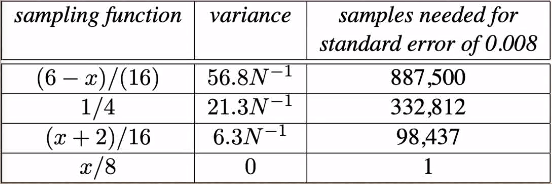如上图所示，这里选用了如第一列所示的4种pdf，从第三列的降低到一定误差所需的采样数量可以看到，第一种的pdf最差，最后一种pdf最好，仅需要1个sample就可以达到要求。那么从第一种到第四种发生了什么变化呢？
不难看出pdf的图像是越来越接近被积函数$f(x)$的图像的，也就是说只要pdf越接近$f(x)$收敛速度也就会更快，在极端条件下当$pdf = cf(x)$，即pdf完全正比于被积函数时，方差达到0，此时只需要1个sample就能得到积分的正确结果。我们可以简要的证明一下：
\begin{aligned} V[\frac{1}{N} \sum_{i=1}^{N} \frac{f\left(X_{i}\right)}{pdf\left(X_{i}\right)}] &= \frac{1}{N} V[\frac{f\left(x\right)}{pdf\left(x\right)}]\\ &= \frac{1}{N} V[\frac{f\left(x\right)}{cf(x)}]\\ &=0 \end{aligned}
以上的简单例子其实也基本带出了重要性采样的思想，也就是选用好的pdf来作为采样的分布，而所谓好的pdf也就是 $pdf(x)\propto f(x)$。我们也可以从直观的角度来考虑一下这个问题，假设被积函数图像如下: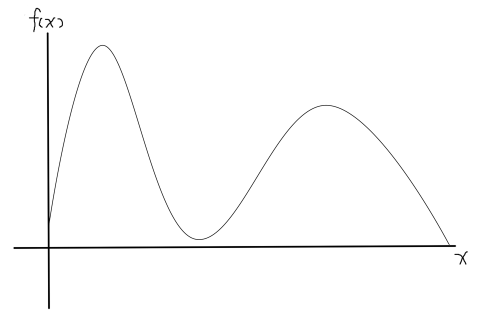当某一区域函数值比较大的时候，这部分积分的值自然会对最终结果有比较大的(重要)影响，理所应当的应该在这部分区域采样更多的点，来减小误差，也就是说f(x)大,pdf(x)也应该大。反之当某一区域函数值比较小的时候，这部分积分的值对最终结果没有比较明显的贡献，因此对于这部分区域减少采样点，即使相对误差较大，但最终放到整体的积分结果中也是可以接受的。综上为什么应该尽量选取$pdf(x)\propto f(x)$也就不难理解了。
2.2 路径追踪中的重要性采样
在理解了重要性采样的想法之后，应该回到路径追踪当中的积分问题，看看如何进行提升：
$L_{o}\left(p, \omega_{o}\right) \approx \frac{1}{N} \sum_{i=1}^{N} \frac{L_{i}\left(p, \omega_{i}\right) f_{r}\left(p, \omega_{i}, \omega_{o}\right)\left(n \cdot \omega_{i}\right)}{p\left(\omega_{i}\right)}$
仔细观察上式，显然积分函数$f(\omega_i) = L_{i}\left(p, \omega_{i}\right) f_{r}\left(p, \omega_{i}, \omega_{o}\right)\left(n \cdot \omega_{i}\right)$. 根据2.1节中所说最佳的采样pdf应该正比于被积函数f(x)，但是这在实际中往往是不可能的，因为$pdf(x) = cf(x)$需要提前知道所有关于f(x)的信息，就比如你想算$L_i$其实本身就是另一个积分问题了。
虽然没有办法得到最优的pdf，但是另一个被广泛采纳的策略是让pdf与被积函数中的一部分成正比，如下图：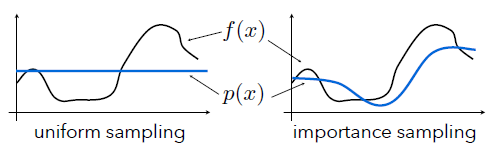在这个例子中左边使用了均匀采样，右边使用了正比于部分被积函数的重要性采样方法，不难想象，右边的收敛速度一定是快于左边的。再回到被积函数：
$f(\omega_i) = L_{i}\left(p, \omega_{i}\right) f_{r}\left(p, \omega_{i}, \omega_{o}\right)\left(n \cdot \omega_{i}\right)$
想要正比于部分被积函数，无非3个选项：

BRDF
incident radiance
cosine term

但$L_i$项在这里的条件下并不可行，所以我们只去介绍如何对cosine项和BRDF进行重要性采样的方法。
2.2.1 Cosine-weighted 半球采样
对cosine项进行重要性采样，也就意味着pdf正比于cosine项，有$pdf(\omega_i) = c * cos\theta_i$，则：
\begin{aligned} & \int_{\Omega^{+}} pdf(\omega) \mathrm{d} \omega = 1\\ & \int_{\Omega^{+}} c * cos\theta_i \mathrm{d} \omega = 1 \end{aligned}
这里省去计算过程，不难得到$c = \frac{1}{\pi}$，利用与第一章类似的方法，再将$d\omega = sin\theta d\theta d\phi$代入，可得：
$pdf(\theta,\phi) = \frac{cos\theta sin\theta}{\pi}$
再计算出边缘概率密度和条件概率密度，以及他们的分布函数：
\begin{aligned} &p d f(\theta)=\int_{0}^{2 \pi} p(\theta, \phi) d \phi=sin2\theta\\ &p d f(\phi \mid \theta)=\frac{p d f(\theta, \phi)}{p d f(\theta)}=\frac{1}{2 \pi}\\ &F(\theta)=\int_{0}^{\theta} \sin2 t d t=1-\cos^2 \theta\\ &F(\phi \mid \theta)=\int_{0}^{\phi} \frac{1}{2 \pi} d t=\frac{\phi}{2 \pi} \end{aligned}
设$\xi_{1}, \xi_{2}$是[0, 1]均匀分布的随机数，利用逆分布函数可以得到采样结果：
$\theta=\cos ^{-1} \sqrt{(1-\xi_{1})}, \phi=2 \pi \xi_{2}$
转化为3维坐标：
$\left\{\begin{array}{l} x=\sin \theta \cos \phi=\cos \left(2 \pi \xi_{2}\right) \sqrt{\xi_{1}} \\ y=\sin \theta \sin \phi=\sin \left(2 \pi \xi_{2}\right)\sqrt{\xi_{1}} \\ z=\cos \theta=\sqrt{1 - \xi_{1}} \end{array}\right.$
最后将cosine-weighted的重要性采样和均匀采样一起运用在环境光遮蔽的计算之中进行比较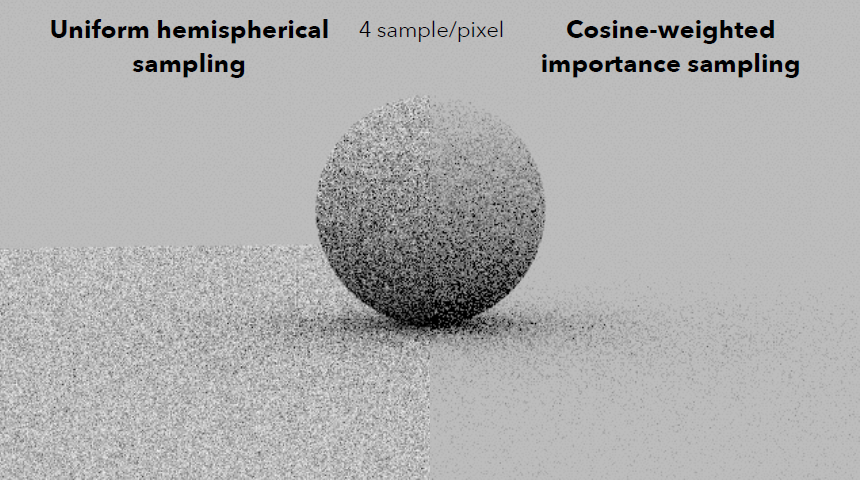可以明显的看出同样是4 samples per pixel，使用cosine-weighted的重要性采样收敛结果远远好于均匀采样。
2.2.2 BRDF采样
既然要根据BRDF进行采样，那么首先自然是确定BRDF的形式，这里采用基于微表面模型理论的BRDF：
$f_{r}\left(\omega_{i}, \omega_{o}\right)=\frac{k_{d}}{\pi}+k_{s} \frac{D\left(\omega_{h}\right) F\left(\left(\omega_{h} \cdot \omega_{i}\right)\right) G\left(\omega_{i}, \omega_{o}, \omega_{h}\right)}{4 \cos \theta_{i} \cos \theta_{o}}, \omega_{h}=\frac{\left(\omega_{i}+\omega_{o}\right)}{\left\|\omega_{i}+\omega_{o}\right\|_{2}}$
其中：
\begin{aligned} &k_{d} \in[0,1]^{3}, 漫反射系数\\ &k_{s}=1-\max \left(k_{d}\right)\\ &F 是菲涅尔项，D为法线分布函数，G为几何函数\\ \end{aligned}
(为了后序推导的方便，这里对微表面BRDF还进行了一定的简化，即没有用菲涅尔项带来计算反射与折射的百分比，而是直接使用kd和ks，简单的保证了能量守恒)
具体来说，这里采用Beckmann分布作为法线分布函数($\theta_h$为微平面法线与宏观法线的夹角)：
$D\left(\omega_{h}\right)=\frac{e^{\frac{-\tan ^{2} \theta_{h}}{\alpha^{2}}}}{\pi \alpha^{2} \cos ^{4} \theta_{h}}$
几何函数则使用Smith近似：
$\begin{array}{c} G\left(\omega_{i}, \omega_{o}, \omega_{h}\right)=G_{1}\left(\omega_{i}, \omega_{h}\right) G_{1}\left(\omega_{o}, \omega_{h}\right) \\ G_{1}\left(\omega_{v}, \omega_{h}\right)=\chi^{+}\left(\frac{\omega_{v} \cdot \omega_{h}}{\omega_{v} \cdot n}\right)\left\{\begin{array}{ll} \frac{3.535 b+2.181 b^{2}}{1+2.276 b+2.577 b^{2}} & b<1.6 \\ 1 & \text { otherwise } \end{array} b=\left(\alpha \tan \theta_{v}\right)^{-1}, \chi^{+}(c)=\left\{\begin{array}{ll} 1 & c>0 \\ 0 & c \leq 0 \end{array}\right.\right. \end{array}$
再次仔细观察BRDF，不难看出，其中分为左边的漫反射项和右边的镜面反射项，当单独考虑坐标的漫反射项时可以结合原被积函数中的cos项，使其变为一个cosine-weighted的重要性采样。此时有：
$pdf(\omega_i) = \frac{cos\theta}{\pi}$
当不看漫反射项，单独考虑镜面反射项的时候其式子略有一些复杂，不过可以做一做排除法，菲涅尔项需要知道采样方向之后才能计算，所以需要排除在外，几何函数项并非连续函数，所以也可以直接排除在外，那么此时分母上只剩下法线分布函数了，而我们所要做的也正是使得pdf正比于法线分布函数项！回想在前文Cook-Torrance BRDF推导中，曾对法线分布函数的物理含义进行具体的定义，即每单位面积,每单位立体角所有法向为$\omega_h$的微平面的面积，利用该点定义可以推出：
$\int_{\mathcal{H}^{2}} \cos \theta_{h} D\left(\boldsymbol{\omega}_{h}\right) \mathrm{d} \boldsymbol{\omega}_{h}=1$
可以看到积分结果恰好为1，那么被积函数应该是一个概率密度函数，但可惜的是这里是$\omega_h$的概率密度函数，而需要的是$\omega_i$的概率密度函数，所以需要一个转换，同样利用Cook-Torrance BRDF推导文中得到的$d{\omega_i}$和$d{\omega_h}$的关系：
$\frac{d\omega_h}{d\omega_i} = \frac{1}{4(\omega_h \cdot \omega_i)}$
将此式代入：
$\int_{\mathcal{H}^{2}}\frac{ \cos \theta_{h}}{4(\omega_h \cdot \omega_i)} D\left(\boldsymbol{\omega}_{h}\right) \mathrm{d} \boldsymbol{\omega}_{i}=1$
因此最终得到想要的$pdf(\omega_i) = \frac{ \cos \theta_{h}}{4(\omega_h \cdot \omega_i)} D\left(\boldsymbol{\omega}_{h}\right)$。（关于这部分直接拿来用的关系可以参考前文推导，这里就不重复推一遍了）
有的读者可能会疑惑，绕这么一圈得到单独考虑brdf漫反射项和镜面反射项的重要性采样分布是为了什么，最后不还是只能对单独某一个pdf采样吗。答案是为了尽可能抓住brdf的特性，所以最终采样的pdf不仅仅是漫反射部分，也不仅仅是镜面反射部分，而是把两部分结合起来的一个全新的pdf：
$k_{s} D\left(\omega_{h}\right) \frac{ \cos \theta_{h}}{4(\omega_h \cdot \omega_i)} +\left(1-k_{s}\right) \frac{\cos \theta_{i}}{\pi}$
那么要想符合这个pdf进行重要性采样，需要首先生成一个随机数$r1$，如果$r1$小于ks则用镜面反射部分的pdf来采样，否则则用漫反射部分的pdf进行采样。这种结合的方式其实是非常直观的，如果ks大也就是镜面反射多，那么就应该以更大的概率用镜面反射部分的pdf来采样，反之亦然。
(tips：由于对brdf的简化，所以这里的结合方式不是那么的物理，如果使用更加物理的brdf，这里的结合需要考虑metalness，个人感觉这里的ks应该设为$max(lerp(0.04,\rho,metalness))$，因为找了一些资料也没找到具体的设置方法，所以不保证正确，有大佬知道也欢迎告知)
在2.2.1节，已经推导过了cosine-weighted的重要性采样，所以当$r1>k_s$的时候，直接用那一套的方法就可以了，但是当$r1时，对于镜面反射部分pdf还不知道如何进行采样，这里给出一个简要的推导：
虽然我们已经得到了对于镜面反射部分$pdf(\omega_i) = \frac{ \cos \theta_{h}}{4(\omega_h \cdot \omega_i)} D\left(\boldsymbol{\omega}_{h}\right)$。但实际上并不需要对$\omega_i$直接采样，更好的方法是对$pdf(\omega_h) = \cos \theta_{h} D\left(\boldsymbol{\omega}_{h}\right)$直接采样微平面法线，再利用$\omega_o$转换到$\omega_i$即可（因为二者关于微平面法线呈反射关系）。同样的，对于直接采样微平面法线使用的还是在第一章里面所说的那一套方法：
\begin{aligned} & \int_{\Omega^{+}} pdf(\omega_h) \mathrm{d} \omega_h = 1\\ & \int_{\Omega^{+}} pdf(\omega_h) \sin\theta_h\mathrm{d} \theta_h \mathrm{d} \phi_h = 1 \end{aligned}
得到：
$pdf(\theta_h,\phi_h) = \frac{e^{\frac{-\tan ^{2} \theta_h}{\alpha^{2}}}}{\pi \alpha^{2} \cos ^{4} \theta_h} \cos \theta_h \sin \theta_h$
关于边缘概率密度，条件概率密度，以及对应的分布函数，这里就不推导了，主要是过程比较繁杂，但实际上就是积分运算（可以令$x = \cos\theta$，则$\tan ^{2} \theta=\frac{1-x^{2}}{x^{2}}$，来简便推导运算过程）
最终可以得到：
$\theta_h=\tan^{-1} \sqrt{-\alpha^2*\ln(1-\xi_1)} , \phi_h=2 \pi \xi_{2}$
从球极坐标转化为3维坐标之后，别忘了在再用$\omega_o$关于$\omega_h$镜面反射到$\omega_i$即可。
最后，均匀采样v.s.BRDF重要性采样：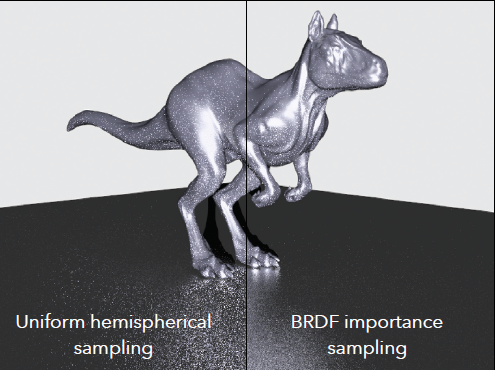注：虽然本文给出的是Beckmann分布作为法线分布函数的BRDF采样，但改成GGX也是一样的推导过程，不同的是积分运算的结果。
3 多重重要性采样的运用
在第二章中介绍了几种重要性采样分布的具体方法，确实都大大加速了收敛的速度，值得一提的是，在讲蒙特卡洛路径追踪的前文中，使用将光照分为直接光照和间接光照的方法（好像英文叫Next Event Estimation，不太懂为啥这样叫）本质上也可以看做是一种重要性采样的方法，因为在计算直接光照的时候是对光源直接采样的。那么是不是单纯的重要性采样已经足够了呢，答案显然是否定的。我们来观察这样一个场景：场景中首先有4个大小不一，颜色不同的光源，悬挂在半空中，其次还有四块长板子，这4块长板有着不同的光滑程度，最底端的最为粗糙，最顶端的最为光滑。
在这样一个情形之下直接对光源采样来计算直接光照得到的就是上图中的结果，可以看到在图片的右上角部分十分的noise。造成这种现象的原因是长板十分光滑，意味着它的brdf接近delta函数（只有一个小范围才可能对计算结果有贡献），而光源又比较大，在这么大一个光源上进行均匀采样的时候，很难恰好找到那一个有贡献的小的范围，而这就造成了越靠近右上角越noise的现象。
既然直接采样光源不行，那就换一种重要性采样，用BRDF采样!即使换了一种采样方法，不难看到在图片的左下角依然出现了非常noise的现象，造成这样的结果的原因是长板十分粗糙，所以用brdf采样的时候其实也就有点接近均匀采样了，那么对于很小的光源几乎就是不可能碰撞到的了。
虽然说直接光源采样不行，BRDF采样也不给力，但重新回顾一下两种重要性采样方法的结果，light采样不行的地方似乎brdf很行，而brdf不行的地方，light又很行，二者形成了一个貌似互补的关系！那么有没有什么好的方法能够将两种重要性采样方法的优点给结合起来呢，这就要提到Veach所提出的大名鼎鼎的多重重要性采样(Multiple Importance Sampling, MIS)方法了。
MIS提供了一种将多种采样分布结合起来的无偏估计的方法，假设现在有$n$种采样分布，每种采样分布采样了$n_i$个点，则最后的估计为：
$F=\sum_{i=1}^{n} \frac{1}{n_{i}} \sum_{j=1}^{n_{i}} w_{i}\left(X_{i, j}\right) \frac{f\left(X_{i, j}\right)}{p_{i}\left(X_{i, j}\right)}$
可以看到其本身依旧是一个蒙特卡洛积分的形式，只不过对于从不同的采样分布中采样的结果都乘上了一个与之对应的权重$w_{i}\left(X_{i, j}\right)$. 要想保证这样的estimator依旧是无偏的则需要满足以下两个条件：
$\begin{array}{l} 1.\sum_{i=1}^{n} w_{i}(x)=1 \text { whenever } f(x) \neq 0, \text { and } \\ 2. \space w_{i}(x)=0 \text { whenever } p_{i}(x)=0 \end{array}$
其中关于第一个条件进一步理解是，只要在$f(x)$有值的地方，就一定存在某个分布能够采样到，从另外一个角度来说某些分布完全可以专门负责某些特殊的地方即可。
对于这样一个estimator，它的无偏性的证明也是比较简单的：
\begin{aligned} E[F] &=\sum_{i=1}^{n} \frac{1}{n_{i}} \sum_{j=1}^{n_{i}} \int_{\Omega} \frac{w_{i}(x) f(x)}{p_{i}(x)} p_{i}(x) dx \\ &=\int_{\Omega} \sum_{i=1}^{n} w_{i}(x) f(x) dx\\ &=\int_{\Omega} f(x) dx \end{aligned}
那么对于这样一个无偏的estimator，该如何设置权重，才能够很好的把各个重要性采样分布的有点结合起来了呢？这里简要介绍两种：
1. Balance heuristic:
$w_{s}(x)=\frac{pdf_{s}(x)}{\sum_{j} pdf_{j}(x)}$
这种设置方法的目的很直接，倘若在一个点你采样的pdf越大，其实也就说明了这个重要性采样分布更加擅长这个区域，理应给它更高的权重，而如果pdf小，意味着该重要性采样分布对这个区域没有什么自信，当然应该减小权重，来降低误差。可能这样说比较抽象，我们就以本章一开始的场景为例子，假设$pdf1$为光源采样,$pdf2$为BRDF采样。
1.当在右上角使用光源采样的时候，由于光源较大，那么$pdf1$自然就会比较小，而BRDF采样由于长板比较光滑，所以$pdf2$会相对较大，综合下来$w_1$就会比较小，降低了在右上角使用光源采样从而造成的误差(想想第一张图的那些右上角noise，现在都会被乘一个小的权重)
2.当在左下角使用BRDF采样的时候，由于光源较小，那么$pdf1$自然就会比较大，而此时长板十分粗糙，所以$pdf2$就比较小，因此$w_2$就会比较小，降低了在左上角使用BRDF采样从而造成的误差(想想第二张图的那些左下角noise，现在都会被乘一个小的权重)
经过以上的解释，相信已经能够大致明白MIS的一个原理了。这里要介绍的第二种权重的设置方法，其实就是在第一种的基础之上用一个指数加大了pdf的差距，使得这种差别变的更加明显
2.Power heuristic：
$w_{s}(x)=\frac{pdf_{s}(x)^{\beta}}{\sum_{j} pdf_{j}(x)^{\beta}}$
在原论文中，建议$\beta = 2$. 在具体实践中，这种进一步扩大pdf区别的power heuristic方法一般可以取得更好的效果。
看看使用了power heuristic的MIS的方法之后：该图片显然结合了光源采样和BRDF采样的优点，不再会在右上角和左下角出现noise了。再看一看该场景之下的权重图：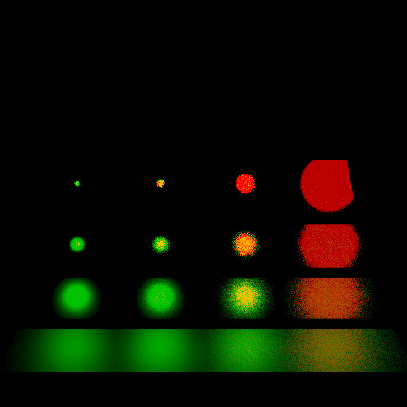红色代表BRDF的权重更大，绿色代表light sampling的权重更大，黄色则代表差不多，回想一下之前的分析，完全符合预期！
最后给出一个实现MIS的伪代码以供参考：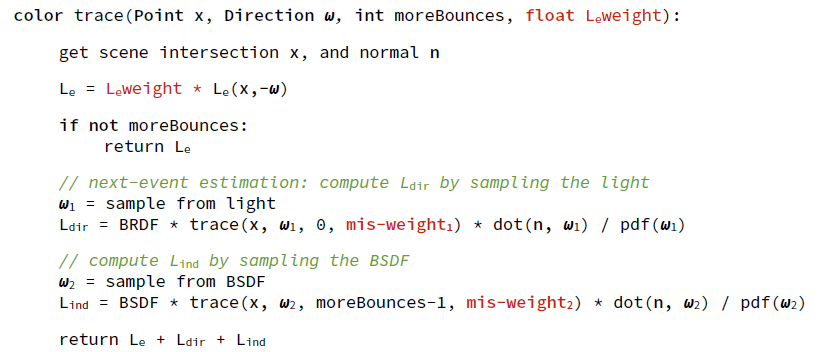该代码可以看作是在NEE的基础之上修改添加了MIS，这里的两个重要性采样分布就是光源采样与BRDF采样。它们是符合本章一开始所讲的那两个条件的，所以结果一定是一个无偏估计！
或者换一种角度看，其实就相当于在计算直接光照的时候有两部分构成，一部分是光源采样，一部分是BRDF采样，它们并不是重复计算，因为都乘了一个weight，被缩小了。而在计算间接光照的时候，实际上还是只有BRDF采样在干活。
总结：
本次文章的内容其实相当于是对前文蒙特卡洛路径追踪的进一步补充，使得这个算法更加的完善，也算是个人的对重要性采样和多重重要性采样的总结，实际上还应该讲一讲低差异序列，也可以进一步减低方差，不过就偷懒了。
另外如果对本文有任何疑问或者发现错误，欢迎大家来指正交流！Refernce：
Veach 97. Robust Monte Carlo Methods for Light Transport Simulation Chapter 9
Multiple Importance Sampling Blog
Dartmouth CS87/187 Slides
Ray Tracing: The Rest of Your Life
Chinagraph2020会前课程3
Nori
chopper:蒙特卡洛积分


展开全文全局光照
• 针对高斯分布策略函数由于截断动作导致算法收敛速度变慢的问题，提出了一种重要性采样优势估计器（ISAE）。该估计器在通用优势估计器（GAE）的基础上，引入了重要性采样机制，通过计算边界动作的目标策略与行动策略...强化学习 深度强化学习
•研究论文
• 重要性采样(IS) 目标：计算服从于target PDF分布的函数f(z)f(z)f(z)的期望，通过应用Monte Carlo方法在概率分布中抽取N个样本，则 E[f(z)]≈1N∑i=1Nf(zi)E[f(z)] \approx\frac{1}{N}\sum_{i=1}^{
Basic Monte Carlo Sampling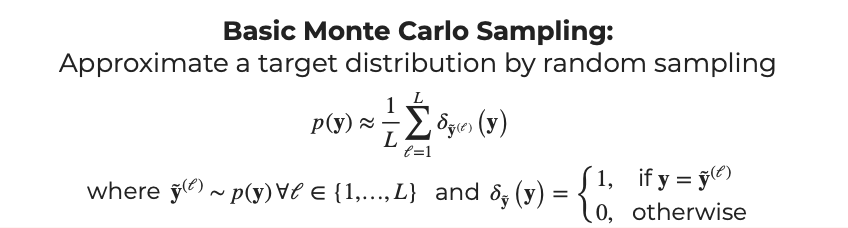应用场所
粒子滤波：针对非线性、非高斯分布的模型，用采到的样本表示概率分布（target PDF），求解$p(z_t | x_{1:t})$（根据1到t时刻的观测变量x求解t时刻隐变量z）
重要性采样(IS)

目标：计算服从于target PDF分布的函数$f(z)$的期望，通过应用Monte Carlo方法在概率分布中抽取N个样本，则
$p(\mathbf{y})=E[f(z)] \approx\frac{1}{N}\sum_{i=1}^{N}f(z_i)$
但如果概率分布比较复杂，则可以通过q(z)（proposal distribution）作为桥梁(importance sampling):
$E[f(z)] = \int_zf(z)p(z)dz = \int_zf(x)\frac{p(z)}{q(z)}dz = \sum_{i=1}^Nf(z_i)\frac{p(z_i)}{q(z_i)}$
直接采样，然后对每个样本应用权重得到期望的近似估计，最后进行权重归一化。

在滤波问题求解$p(z_t | x_{1:t})$时，权重表达式：
$w_t^i = \frac{p(z_{t}^i | x_{1:t})}{q(z_{t}^i| x_{1:t})}$

例子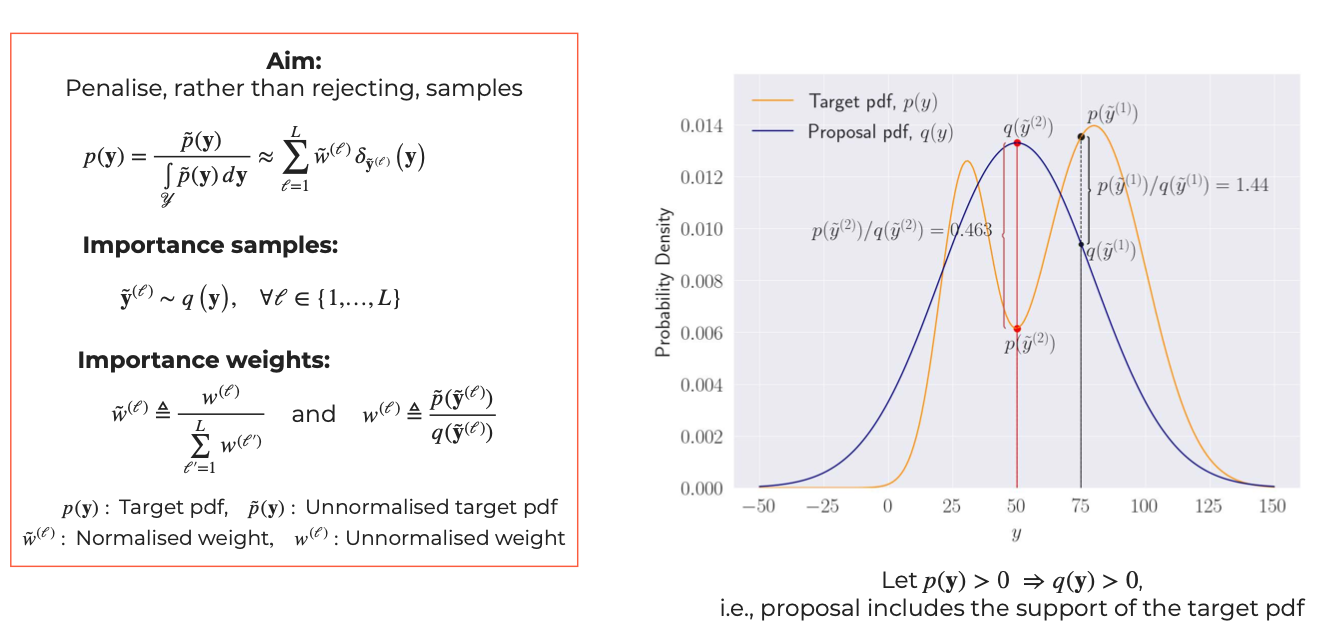先从q分布得到L个样本$\tilde{\mathbf{y}}^{(\ell)}$,然后计算权重$w^{(\ell)}$，最后进行权重归一化得到每个样本对应的权重$\tilde{w}^{(\ell)}$

序列重要性采样(SIS)

求解$p(z_{1:t} | x_{1:t})$
$w_t^i \propto \frac{p(z_{1:t} | x_{1:t})}{q(z_{1:t}| x_{1:t})}$

$p(z_{1:t} | x_{1:t}) \propto p(x_{1:t}, z_{1:t}) =p(x_t |z_{1:t},x_{1:t-1})p(z_{1:t}, x_{1:t-1}) \\=p(x_t | z_t)p(z_t|z_{1:t-1},x_{1:t-1})p(z_{1:t-1},x_{1:t-1}) \\=p(x_t | z_t)p(z_t|z_{t-1})p(z_{1:t-1},x_{1:t-1}) \\ \propto p(x_t | z_t)p(z_t|z_{t-1})p(z_{1:t-1}|x_{1:t-1})$
指定$q(z_{1:t}| x_{1:t})=q(z_{t}|z_{1:t-1}, x_{1:t})q(z_{1:t-1}|x_{1:t-1})$
得到：
$w_t^i \propto \frac{p(z_{1:t} | x_{1:t})}{q(z_{1:t}| x_{1:t})} \propto \frac{p(x_t | z_t)p(z_t|z_{t-1})p(z_{1:t-1}|x_{1:t-1})}{q(z_{t}|z_{1:t-1} x_{1:t})q(z_{1:t-1}|x_{1:t-1})} = \frac{p(x_t | z_t)p(z_t|z_{t-1})}{q(z_{t}|z_{1:t-1} x_{1:t})} w_{t-1}^i$

在t-1时刻采样并计算权重
t时刻根据$q(z_{t}|z_{1:t-1} x_{1:t})$采样得到N个样本$z_t^i$，并计算得到N个权重
权重归一化

SIS 应用于Filtering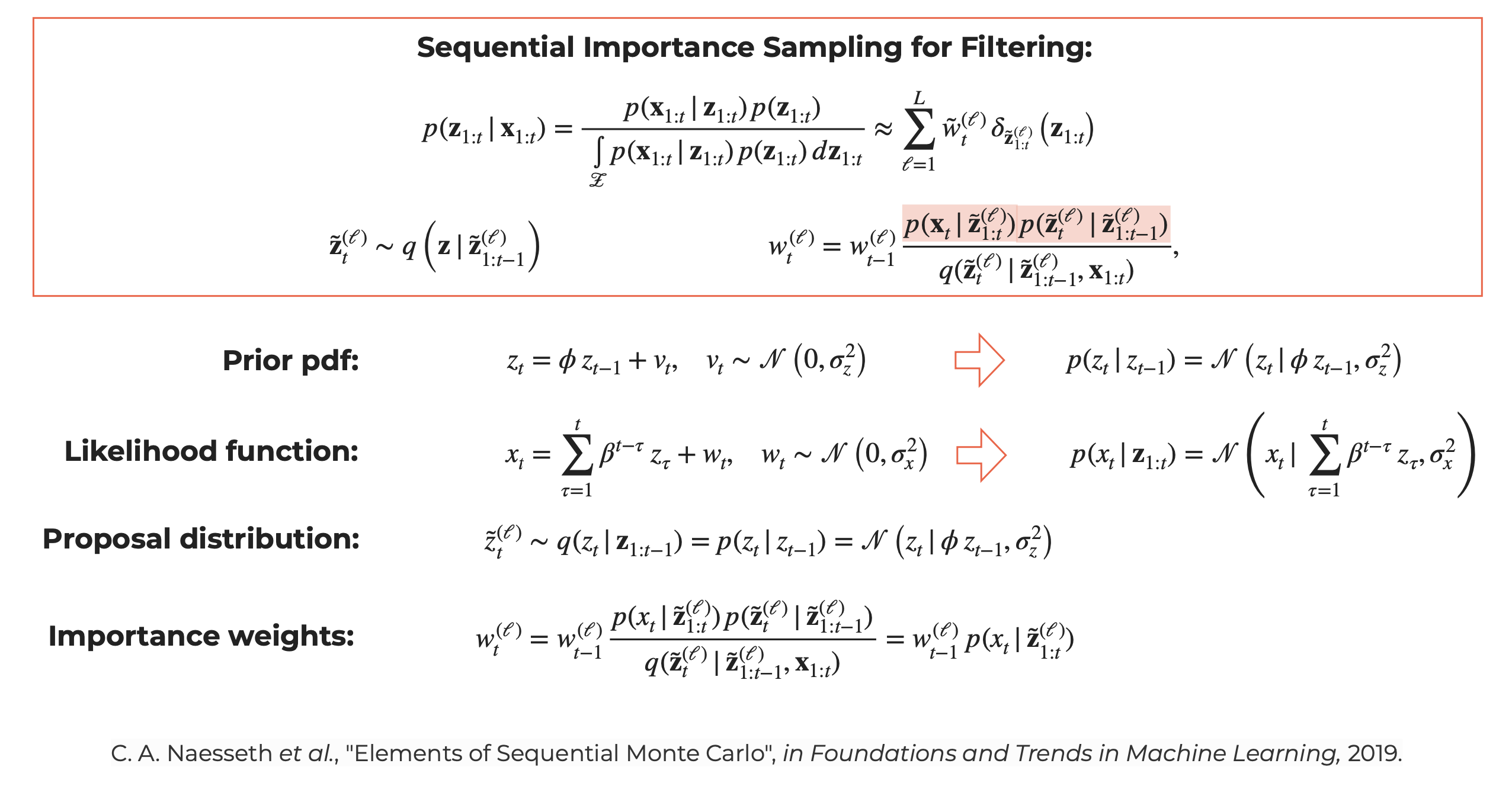通常Filtering问题求解目标为t时刻隐变量的后验$p(z_t | x_{1:t})$，但SIS为了简化运算求解目标为$p(z_{1:t} | x_{1:t})$

根据隐变量z的状态转移方程得到先验分布$p(z_t|z_{t-1})$
根据观测变量x和隐变量z的状态转移方程得到似然函数$p(x_t | z_{1:t})$
设定proposal distribution $q(z)$为隐变量z的先验分布$p(z_t|z_{t-1})$
计算t时刻第$\ell$个样本的权重$w^{(\ell)}$然后进行权重归一化

SIS 存在的问题及改善(SIR)

问题：权重退化
一段时间后，大部分样本的权重会逼近0
加入重采样(Resampling):
Sequential importance resampling(SIR) or
Sequential importance sampling and resampling(SIS/R))：
思路是将权重作为概率分布，得到累计概率密度函数(CDF)，然后在CDF上取点。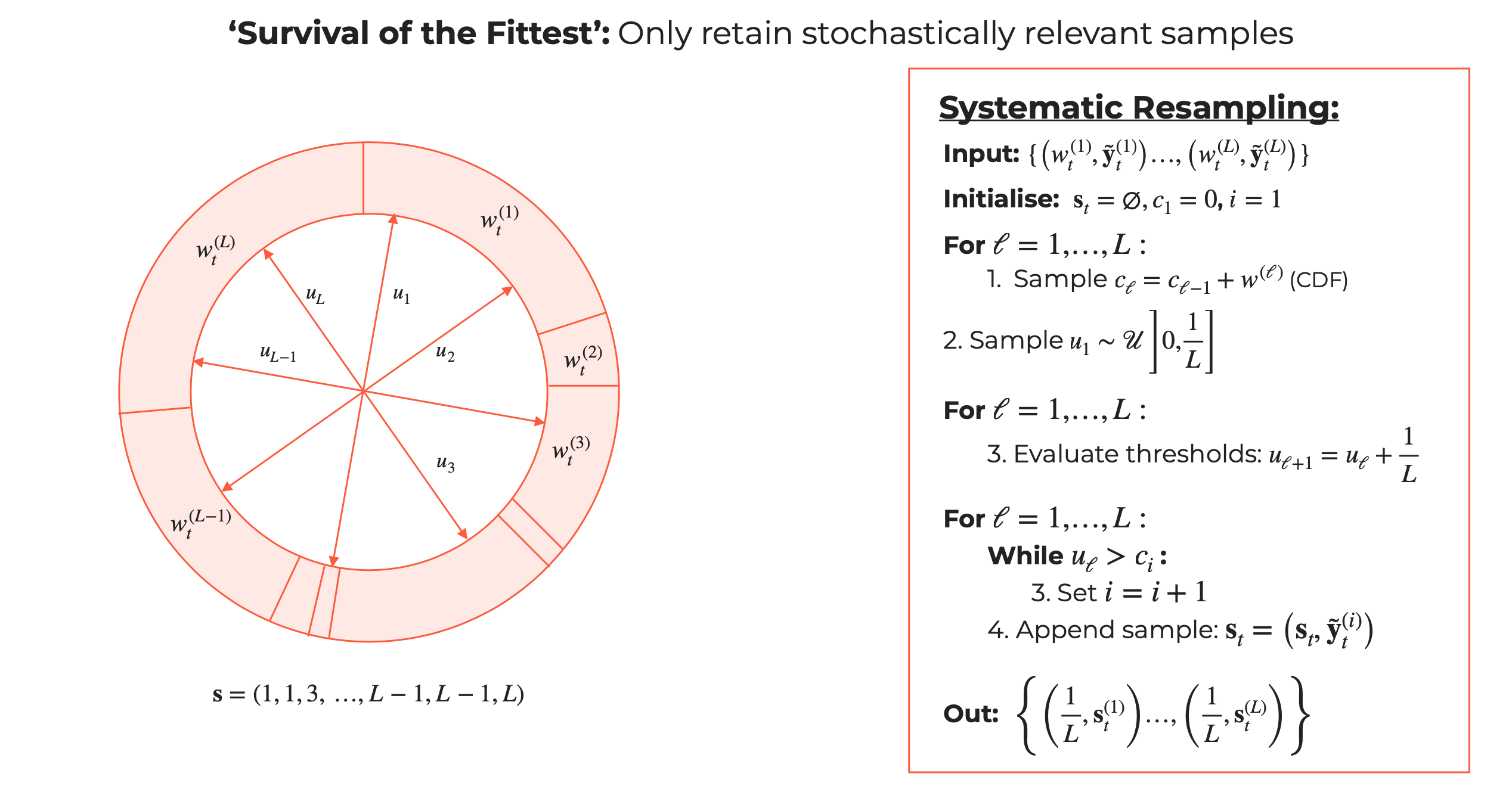算法流程：

输入：
L个SIS产生的$\tilde{\mathbf{y}}^{(\ell)}$和对应的权重$w^{(\ell)}$
初始化：
新的样本集合$s_t$为空
样本权重$w^{(\ell)}$的累积密度函数cdf 的第一个值$c_1$为0。
i为1

计算权重$w^{(\ell)}$的cdf
从(0,$\frac{1}{L}$)区间采样$u_1$
根据$u_1$为L个样本生成对应的$u_{\ell}$
遍历输入样本和权重，如果第${\ell}$个样本的$u_{\ell}$大于$c_i$，则i+1，并将第i个样本放入新的样本集合$s_t$,新的权重为$\frac{1}{L}$

总结：SIR算法为SIS得到样本和权重之后应用重采样，得到新的L个样本，并且每个样本的权重都是$\frac{1}{L}$


展开全文机器学习 强化学习
• 文章转自：...看了好多篇关于采样，重要性采样的文章，很多都有公式与概念的错误，这篇真是最良心的一篇，从头到尾没有发现错误，而且讲得深入浅出，通俗易懂。 引子 最近开始拾起来看一些NLP相关的东...
展开全文• ## 重要性采样简述

千次阅读 2018-06-07 14:12:28
重要性采样（Importance Sampling）是统计学中的一种采样方法。它主要用于一些难以直接采样的数据分布上。假设有一个很复杂的概率密度函数 p(x)p(x)p(x) ，求解随机变量基于此概率下的某个函数期望，即 Ex∼p(x)[f...统计学 强化学习
• 重要性采样在强化学习有着重要作用,它是蒙特卡洛积分的一种采样策略.目录概率论基础蒙特卡洛积分重要性采样参考概率论基础本文先补充两条基础的概率论公式,方便大家更好地看懂全文假设某一连续型随机变量的样本空间...
• 最近在看一篇讲SSR的PPT，里面提到了根据GGX分布做重要性采样，其实之前或多或少的了解过重要性采样算法，不过当时没有认真的总结过这个问题，也没有想过要认真的推导相关的公式。这篇给文章的主要目的就是给看过的...
• 本文讨论一种能很好地改善该问题的技术——多重重要性采样。我们常常需要通过采样来估计某个已知部分结构特征的函数的积分(比如$f(x) = g(x)h(x)$，但函数的具体值却又严重依赖于一组额外的参数，这导致我们很难在...
• Importance Sampling(重要性采样)，也是常用估计函数价值在某个概率分布下的期望的一个方法。这篇博文先简要介绍IS，再将其在策略评估中的应用。
• 重要性采样在强化学习有着重要作用,它是蒙特卡洛积分的一种采样策略. 目录 概率论基础 蒙特卡洛积分 重要性采样 参考 概率论基础 本文先补充两条基础的概率论公式,方便大家更好地看懂全文 假设某一连续型随机...
• 重要性采样（Importance Sampling） 日后补充，统计学相关知识
• 重要性采样主要用于难以直接采样的数据分布上，采样是指从已知的某个分布采样一些数据进行后续运算，但是数据分布比较复杂不容易进行采样，针对这种问题使用蒙特卡罗法，例如： 复杂的概率密度函数p(x)，求解随机...
• ## 理解重要性采样

千次阅读 2015-05-12 21:09:13
重要性采样是非常有意思的一个方法。我们首先需要明确，这个方法是基于采样的，也就是基于所谓的蒙特卡洛法(Monte Carlo)。蒙特卡洛法，本身是一个利用随机采样对一个目标函数做近似。例如求一个稀奇古怪的形状的...
• 文章目录重要性采样(Importance Sampling)透彻学习笔记前言：参考主体：on-policy 和 off-policyOn-policy:Off-policy:重要性采样重要性采样后方差的变化-公式推导 前言： 重要性采样，我在众多算法中都看到的一个...强化学习
• 重要性采样（importance sampling）重要抽样主要为了解决一下几种问题： 1. 为了减小蒙特卡洛方法的方差 2. 为了对很少发生事件（rare event）进行有效采样，这类问题如果用传统蒙特卡洛采样会需要非常大的样本 3. ...
• （1）使用重要性采样时会用什么方法避免pi非零而mu为零的问题？ 一般使用一些平滑算法，例如拉普拉斯一类的平滑 这里beta是平滑的超参数。 还有一种就是clip，也就是ratio如果绝对值超过了某个阈值a，那就设置成这...强化学习 机器学习 人工智能 算法
• 重要性采样是统计学习中一种常用的方法。在强化学习中通常和蒙特卡洛方法结合使用。 重要性采样是，使用另外一种分布来逼近所求分布一种方法。 具体形式是这样的：假设我们在想要求取目标分布PPP下函数f(x)f(x)f(x)...
• 重要性采样在通信系统仿真中的应用，党笑，，重要性采样是一种高效的仿真技术，它的目的在于降低一个既定的估计器的方差，以能在较短时间内得到给定精度的仿真结果。在通信系
• 目录11.1 Basic Sampling AlgorithmsP526 标准概率分布P528 拒绝采样P530 可调节的拒绝采样Adaptive rejection samplingP532 重要性采样P534 采样-重要性-重采样 Sampling-importance-resamplingP536 采样和EM算法P...机器学习 人工智能
• 刘浚嘉：PR：机器人学的概率方法学习路径​zhuanlan.zhihu.comPR 采样分章 第一节：蒙特卡洛采样、重要性采样引言还记得我们之前学过的贝叶斯推断吗？刘浚嘉：PR Ⅱ：贝叶斯估计/推断及其与MAP的区别​zhuanlan....
• 重要性采样在抽样预测领域的应用引言内容组织抽样预测解析抽样流程及核心概念预测目标点预测置信区间（Confidence interval）置信度（Confidence level）抽样*样本数量确定样本抽取标题重要性采样（Importance ...机器学习
• 蒙特卡洛方法、接受拒绝采样、重要性采样、MCMC方法 文章目录一、蒙特卡洛方法1. 什么是蒙特卡洛方法2. 蒙特卡洛积分推导3.python实例承上启下二、接受-拒绝采样1. 核心思想介绍2. 例子三、重要性采样1. 普通重要性...MCMC方法...